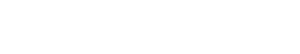# Binomial

Binomial is used to refer to a two-category classification problem. That is, given a set of documents, the goal is to classify each document into one of two mutually exclusive classes.

Binomial may also be used outside of the text analytics industry, where it typically refers to a statistical test that is used to compare the proportions of two groups.

The assumption underlying the binomial test is that the two groups are independent and that each observation can be classified into one of two mutually exclusive categories.

## Binomial vs. Other Terms

Binomial is sometimes used interchangeably with other terms, such as binary and two-class.

However, there are some subtle differences between these terms.

Binary typically refers to a data type that can only take on two values, such as 0 or 1.

Two-class, on the other hand, typically refers to a classification problem with

## Binomial Distribution

Binomial Distribution is the probability distribution of a sequence of independent and identically distributed Bernoulli trials.

A Bernoulli trial is a random experiment with two possible outcomes, which we will label “success” and “failure”.

The Binomial Distribution gives us the probabilities of various numbers of successes in a given number of Bernoulli trials.

## How to solve Binomial Distributions

Binomial Distributions can be solved using a variety of methods, including the use of tables, software, or calculators.

Tables are one way to solve for binomial probabilities.

To use a table, you will need to know the number of trials (n) and the probability of success (p). The formula used to calculate binomial probabilities is:

P(x) = P(X = x) = C(n,x)px(1-p)n-x

Where:

P(x) is the probability of x successes in n trials,

C(n,x) is the number of combinations of n things taken x at a time,

p is the probability of success on a single trial, and

1-p is the probability of failure on a single trial.

## Binomial Limitations

The Binomial Distribution has some limitations.

First, it assumes that the trials are independent, which is not always the case in real-world situations.

Second, it only applies to situations where there are two possible outcomes (success or failure).

If there are more than two possible outcomes, then a different distribution must be used.Unlock the power of actionable insights with AI-based natural language processing.